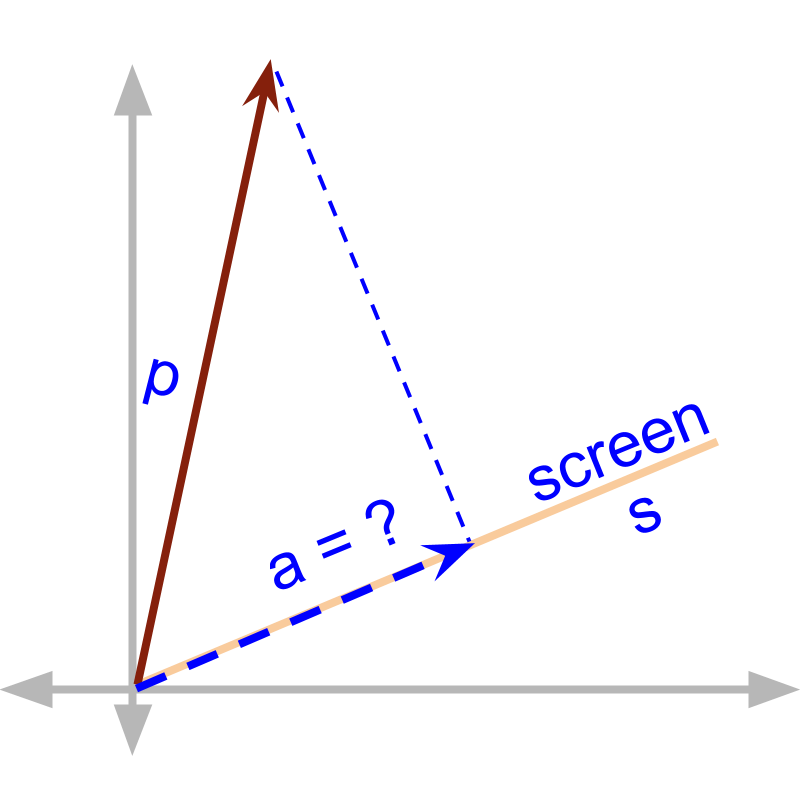maths > vector-algebra

Cross Product with Direction removed

what you'll learn...

Overview

Cross Product With Direction Removed

»  By definition, cross product is a vector

»  Some applications may specify a scalar in the multiplication of components in perpendicular. In such cases, the direction is removed from the vector cross product.

exampleAn object by position vector $\stackrel{\to }{p}$$\vec{p}$ is at a distance from a screen. The screen is given by unit vector $\stackrel{\to }{s}$$\vec{s}$. The distance of the object from the screen is a vector that terminates at the object and at ${90}^{\circ }$${90}^{\circ}$ angle to the screen.

Note: In physics - Kinematics, distance and displacement are defined as scalar and vector. In coordinate geometry, distance from an object to another is a vector.

Given $\stackrel{\to }{p}$$\vec{p}$ and the screen $\stackrel{^}{s}$$\hat{s}$. The magnitude of distance of object from the screen is $|\stackrel{\to }{p}×\stackrel{^}{s}|$$| \vec{p} \times \hat{s} |$ $=|p|\mathrm{sin}\theta$$= | p | \sin \theta$

An object by position vector $\stackrel{\to }{p}$$\vec{p}$ is at a distance from a screen. The screen is given by unit vector $\stackrel{\to }{s}$$\vec{s}$ We saw that the distance is a vector and the length of the distance is $|\stackrel{\to }{p}×\stackrel{^}{s}|$$| \vec{p} \times \hat{s} |$. The direction of the distance vector is 'unit vector along $\stackrel{\to }{p}-\left(\stackrel{\to }{p}\cdot \stackrel{^}{s}\right)\stackrel{^}{s}$$\vec{p} - \left(\vec{p} \cdot \hat{s}\right) \hat{s}$'.

Another Example

The magnetic field is given by $\stackrel{\to }{B}$$\vec{B}$ (a vector quantity).

The velocity of the wire moving in the magnetic field is $\stackrel{\to }{v}$$\vec{v}$ (a vector quantity).

The EMF (electro-magnetic force or the voltage) induced in the wire of length $L$$L$ is given by

$\text{EMF}=L|\stackrel{\to }{v}×\stackrel{\to }{B}|$$\textrm{E M F} = L | \vec{v} \times \vec{B} |$

Note that the EMF is a scalar quantity.

In this, $\stackrel{\to }{v}$$\vec{v}$ has component parallel and another component perpendicular to the magnetic field $\stackrel{\to }{B}$$\vec{B}$. Only the perpendicular component interacts with the magnetic field and results the EMF. That is the reason, the equation has cross product.

The created EMF is by definition a scalar quantity. So, the magnitude of the cross product is used.

summary

Distance using Cross Product: For a vector $\stackrel{\to }{p}$$\vec{p}$ and a direction given by unit vector $\stackrel{^}{s}$$\hat{s}$, the magnitude of distance of point given by $\stackrel{\to }{p}$$\vec{p}$ to the direction $\stackrel{^}{s}$$\hat{s}$ is $|\stackrel{\to }{p}×\stackrel{^}{s}|$$| \vec{p} \times \hat{s} |$.

Vector cross product, by definition, is a vector. Depending on the application requirement, the direction can be removed.

Outline